## Typed Tagless Final Bioinformatics

Sebastien Mondet (@smondet).

C◦mp◦se :: Conference, Thursday, May 18, 2017.

### Context

Seb: Software Engineering / Dev Ops at the Hammer Lab.### Context

Was here 2 years ago to present:

• Ketrew: a workflow engine for complex computational pipelines.
• EDSL/library to write programs that build workflows/pipelines
• A separate application, The “Engine”, orchestrates those workflows
• Biokepi: a library of Ketrew “nodes” for Bioinformatics.

Now

• Used with GCloud/Kubernetes, AWS, YARN (incl. Spark).
• `Tyxml_js` + `react` WebUI
• Personalized Genomic Vaccine clinical trial (NCT02721043) → `hammerlab/epidisco/`

### WebUI ⇒ 3.6 MB GIFs### In Particular, We Presented:

Cool experiment: GADT-based, very high-level pipeline EDSL.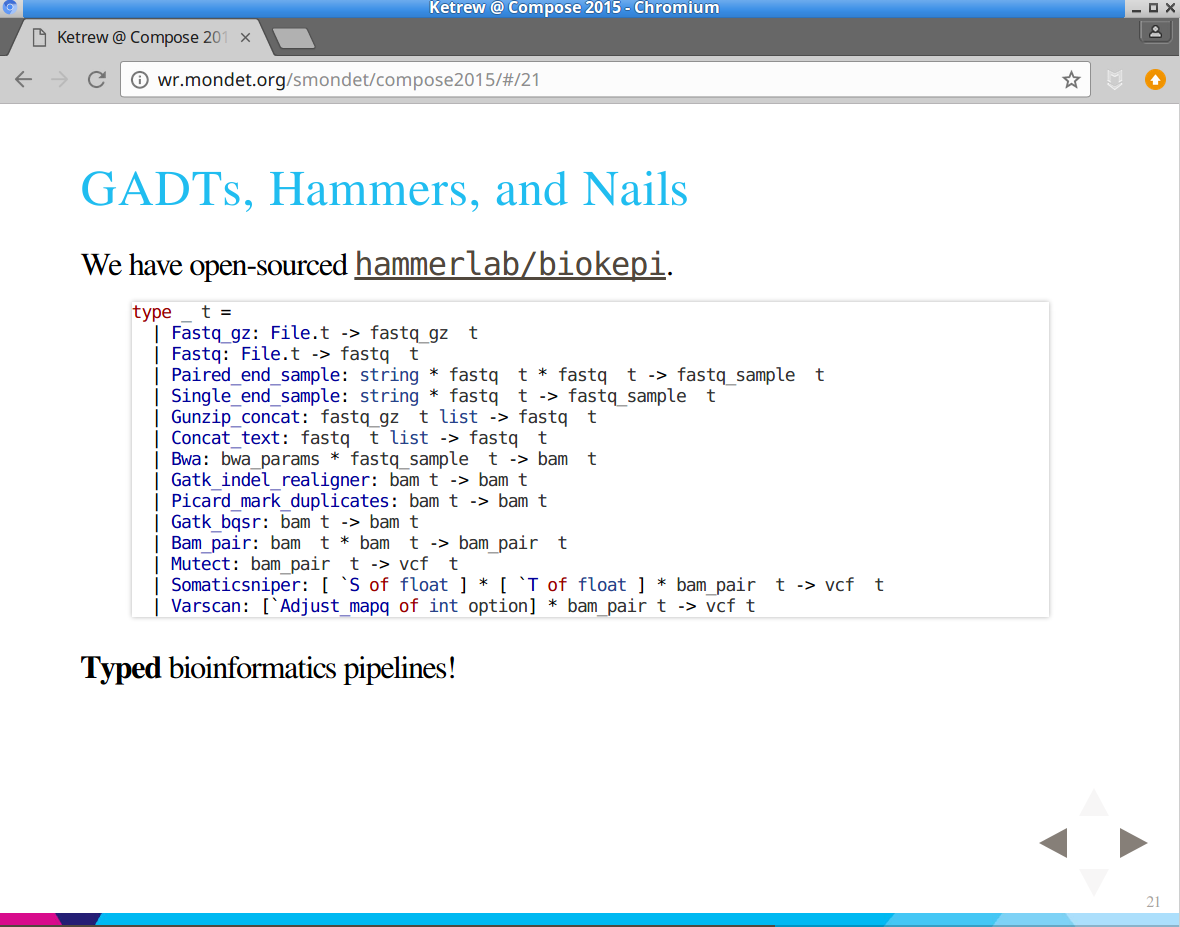### Then, At OCaml / ICFP 2015

Cool experiment: add tools / tool-kinds: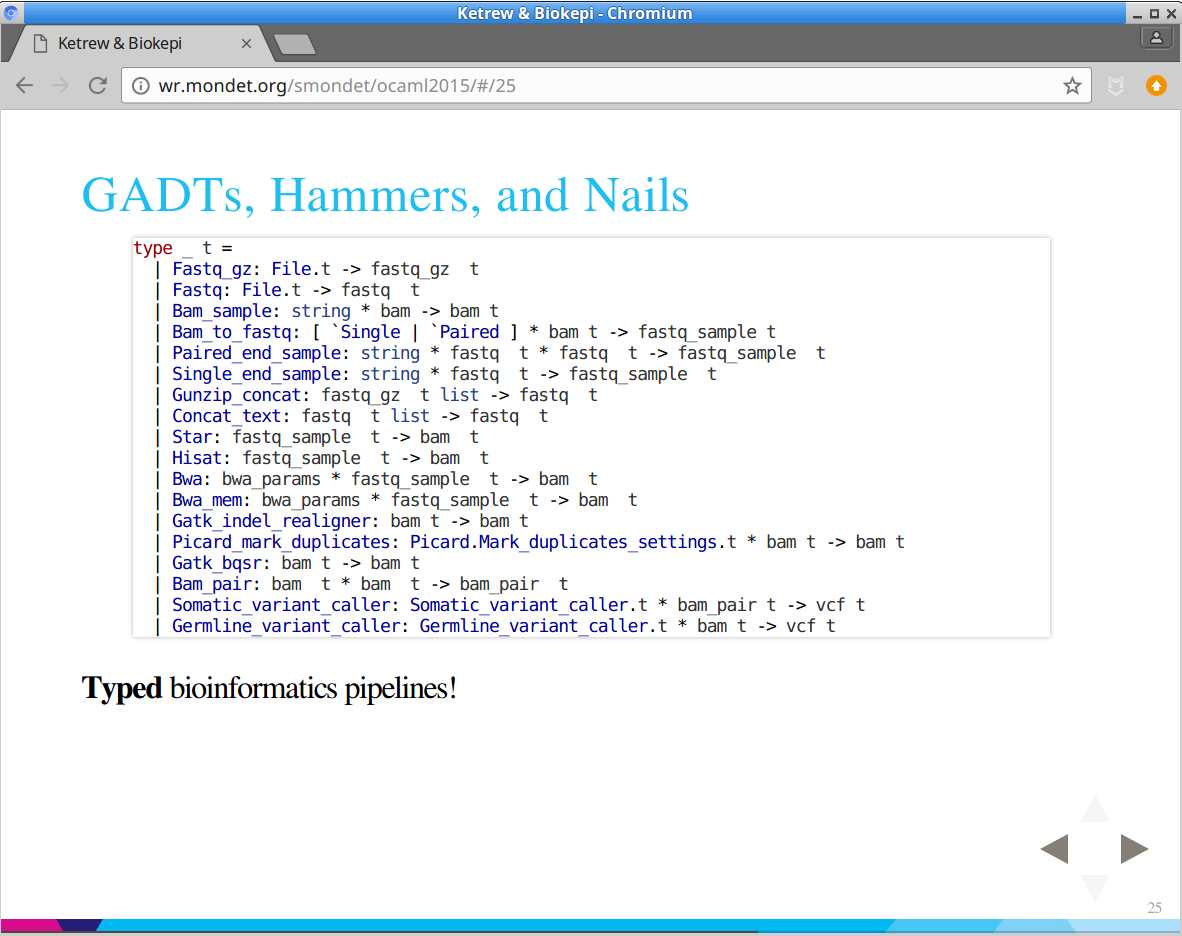### And Soon After

Kept growing, became the default…

```type _ t =
| Fastq_gz: File.t -> fastq_gz  t
| Fastq: File.t -> fastq  t
| Bam_sample: string * bam -> bam t
| Bam_to_fastq: [ `Single | `Paired ] * bam t -> fastq_sample t
| Paired_end_sample: fastq_sample_info * fastq t * fastq t -> fastq_sample t
| Single_end_sample: fastq_sample_info * fastq t -> fastq_sample t
| Gunzip_concat: fastq_gz t list -> fastq t
| Concat_text: fastq t list -> fastq t
| Star: Star.Configuration.Align.t * fastq_sample t -> bam t
| Hisat: Hisat.Configuration.t * fastq_sample t -> bam t
| Stringtie: Stringtie.Configuration.t * bam t -> gtf t
| Bwa: Bwa.Configuration.Aln.t * fastq_sample t -> bam t
| Bwa_mem: Bwa.Configuration.Mem.t * fastq_sample t -> bam t
| Mosaik: fastq_sample t -> bam t
| Gatk_indel_realigner: Gatk.Configuration.indel_realigner * bam t -> bam t
| Picard_mark_duplicates: Picard.Mark_duplicates_settings.t * bam t -> bam t
| Gatk_bqsr: (Gatk.Configuration.bqsr * bam t) -> bam t
| Bam_pair: bam t * bam t -> bam_pair t
| Somatic_variant_caller: somatic Variant_caller.t * bam_pair t -> vcf t
| Germline_variant_caller: germline Variant_caller.t * bam t -> vcf t
| Seq2HLA: fastq_sample t -> seq2hla_hla_types t
| Optitype: ([`DNA | `RNA] * fastq_sample t) -> optitype_hla_types t

### Very Concise Pipelines

```let crazy_example ~normal_fastqs ~tumor_fastqs ~dataset =
let open Pipeline.Construct in
let normal = input_fastq ~dataset normal_fastqs in
let tumor = input_fastq ~dataset tumor_fastqs in
let bam_pair ?gap_open_penalty ?gap_extension_penalty () =
let normal =
bwa ?gap_open_penalty ?gap_extension_penalty normal
|> gatk_indel_realigner |> picard_mark_duplicates |> gatk_bqsr in
let tumor =
bwa ?gap_open_penalty ?gap_extension_penalty tumor
|> gatk_indel_realigner |> picard_mark_duplicates in
pair ~normal ~tumor in
let bam_pairs = [
bam_pair ();
bam_pair ~gap_open_penalty:10 ~gap_extension_penalty:7 ();
] in
let vcfs =
List.concat_map bam_pairs ~f:(fun bam_pair ->
[
mutect bam_pair;
somaticsniper bam_pair;
somaticsniper ~prior_probability:0.001 ~theta:0.95 bam_pair;
varscan_somatic bam_pair;
strelka ~configuration:Strelka.Configuration.exome_default bam_pair;
])
in
vcfs```

### Type Information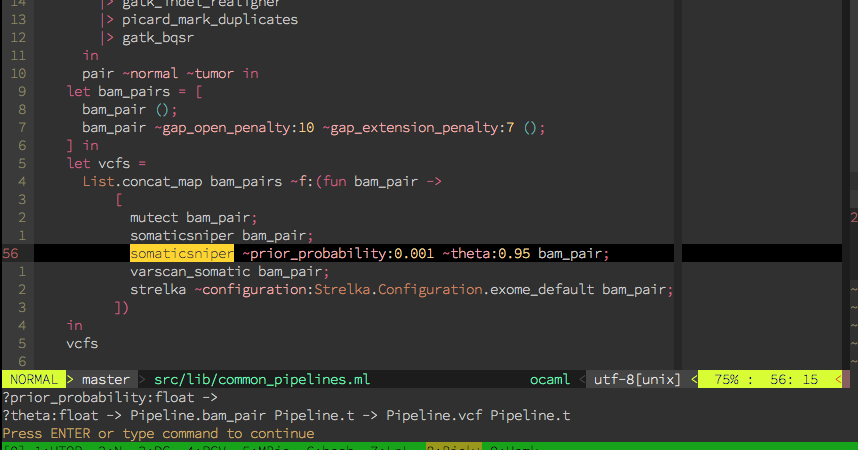### There's a “But”

Fancy but not that practical:

• `Pipeline.t` is getting too big
• Just `compile_aligner_step` is about 170 lines of pattern-matching
• Still missing proper `lambda`/`apply`, list functions, etc.
• Not Extensible
• Adding new types is pretty annoying.
• Optimization passes need to deal with whole language at once, always.
• Optimization are not proper language transformations.

### Try Again

We want what we already have + users of the library to be able to:

• Extend the language to their needs
• Re-use default compilers when implementing theirs
• Write future-proof optimizations
• Do transformations “by hand” if easier than an optimization pass

### Not-Really Extensible Hacks

Tried a few experiments:

• extensible types
• loose a lot of the type-strength benefits
• are not that extensible
• basic “language” based-on GADTs and extensible bioinformatics atoms
• could have worked further but not really extensible either

### Oleg

“We trivially and elegantly solved that problem 20 years ago!”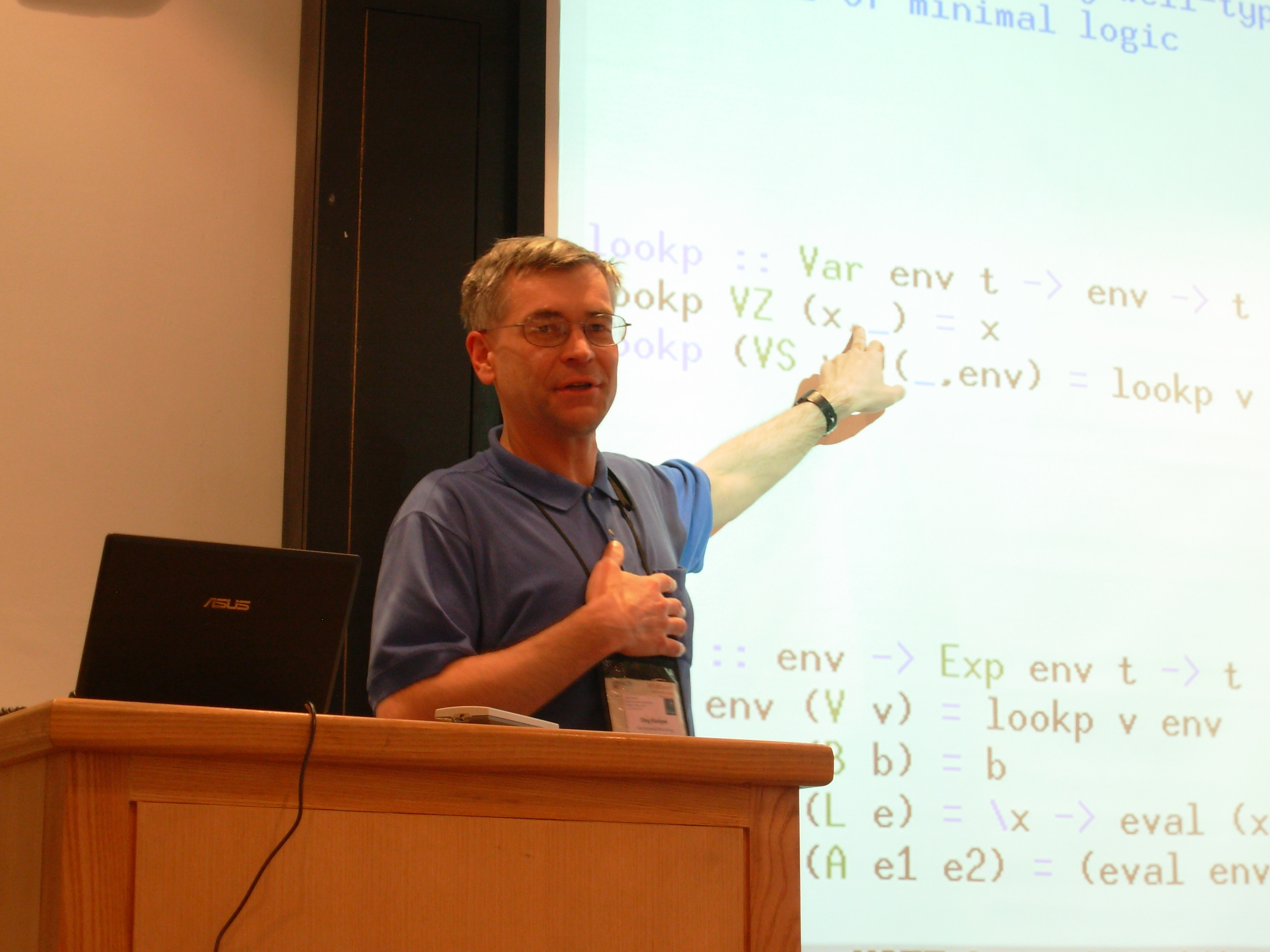### QueΛ and The Course Notes

First:

@pveber pointed us to Oleg's course:

• In Haskell (very concise code, very un-modular).
• Well explained and progressive.

⇒ Follow the course; with QueΛ's help; in a Biokepi-like setting.

### And We Did It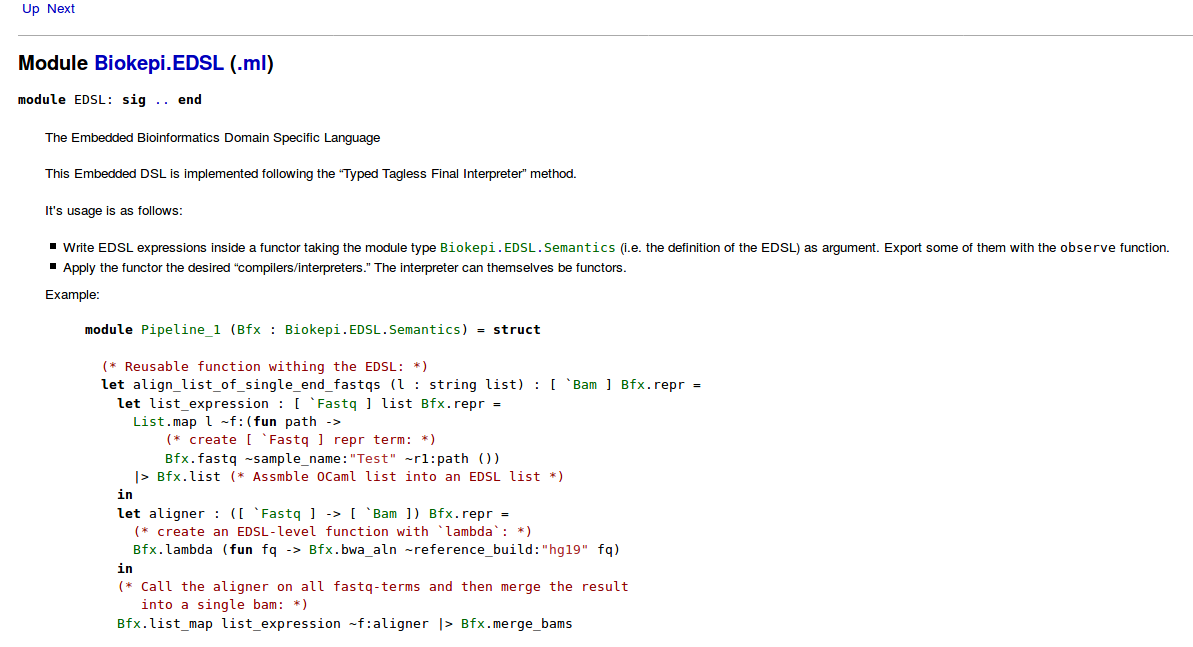### We TTFI-ed Everything

And it's more powerful:

• More constructs: `lambda`/`apply`, list and pair functions, …
• Easier to document.
• Easier to maintain.
• Extensible by the users.

And keeps growing:

`````` \$ grep 'val ' src/pipeline_edsl/semantics.ml | wc -l
56``````

### How Does It Work?

Now tutorial mode:

• Translation to TTFI.
• Show how to manipulate the pseudo-AST.
• Show how to extend the EDSL.

Type Constraints + Existential Types:

```type _ t =
| Int: int -> int t
| True: bool t
| False: bool t
| Equal: 'a t * 'a t -> bool t

let rec eval: type v. v t -> v =
function
| Int i -> i
| True -> true
| False -> false
| Equal (a, b) -> (=) (eval a) (eval b)

let () = assert (eval (Int 42) = 42)
let () = assert (eval (Equal (True, (Equal (Int 42, Int 42)))) = true)```

### TTFI

Type Constraints + Existential Types, using module types and functors:

```module type Symantics = sig
type 'a repr
val int: int -> int repr
val t: bool repr
val f: bool repr
val equal: 'a repr -> 'a repr -> bool repr
end

module Eval_ocaml : Symantics with type 'a repr = 'a = struct
type 'a repr = 'a
let int i = i
let t = true
let f = false
let equal a b = (a = b)  (* Cheating a bit *)
end

module Examples (EDSL: Symantics) = struct
let ex1 = EDSL.int 42
let ex2 = EDSL.(equal t (equal (int 42) (int 42)))
end

let () =
let module Compiled_examples = Examples(Eval_ocaml) in
assert (Compiled_examples.ex1 = 42);
assert (Compiled_examples.ex2 = true);
()```

### TTFI in Bullet Points

In OCaml:

• defintion of the language: `module type Semantics`
• program: `functor: Semantics -> whatever`
• compiler: `module implementing Semantics`
• optimization/transformation: `functor: Semantics -> Semantics`
• optimization framework: functor + GADT that implements “default behavior”

### Mysteriously Useful Bit

More jargon: “observations” are useful artifacts of optimization passes:

```module type Symantics = sig
type 'a repr
val int: int -> int repr
val t: bool repr
val f: bool repr
val equal: 'a repr -> 'a repr -> bool repr
type 'a observation
val observe: (unit -> 'a repr) -> 'a observation
end```

### To-String Compiler

```module Eval_string
: Symantics with type 'a repr = string and type 'a observation = string
= struct
type 'a repr = string
let int = string_of_int
let t = "True"
let f = "False"
let equal a b = Printf.sprintf "(%s = %s)" a b
type 'a observation = string
let observe f = f ()
end
module More_examples (EDSL: Symantics) = struct
let ex1 =
let open EDSL in
observe (fun () -> int 42)
let ex2 =
let open EDSL in
observe (fun () ->
equal (equal t t) (equal (int 42) (int 43))
)
end
let () =
let module Compiled_examples = More_examples(Eval_string) in
Printf.printf "Ex1: %s\nEx2: %s\n%!"
Compiled_examples.ex1 Compiled_examples.ex2;
()```
``````Ex1: 42
Ex2: ((True = True) = (42 = 43))``````

### Simple Optimization Example

We can do some rewriting with functors:

```module True_equal_true_true (Input: Symantics)
: Symantics with type 'a observation = 'a Input.observation
= struct
include Input
let t = Input.(equal t t)
end

let () =
let module Compiled_examples = More_examples(True_equal_true_true(Eval_string)) in
Printf.printf "Ex1: %s\nEx2: %s\n%!"
Compiled_examples.ex1 Compiled_examples.ex2;
()```
``````Ex1: 42
Ex2: (((True = True) = (True = True)) = (42 = 43))``````

(this works without the `'a observation` thing …)

### Not Enough

For more complex/interesting transformations,
what we really want is to `match term with`:

```type _ t =
| Int: int -> int t
| True: bool t
| False: bool t
| Equal: 'a t * 'a t -> bool t

let rec transform_equal_true_true : type v. v t -> v t =
function
| Int i -> Int i
| True -> True
| False -> False
| Equal (True, True) -> True (* Optimization Pass ! *)
| Equal (a, b) ->
Equal (transform_equal_true_true a, transform_equal_true_true b)

let () =
assert (
transform_equal_true_true (Equal (False, (Equal (True, True))))
=
(Equal (False, True))
)```

### Optimization Framework

Some type-hackery later … A Generic Extensible Optimization Pass Generator.

```module type Transformation_base = sig
type 'a from
type 'a term
val fwd : 'a from -> 'a term (* reflection *)
val bwd : 'a term -> 'a from (* reification *)
end
module Generic_optimizer
(X: Transformation_base)
(Input: Symantics with type 'a repr = 'a X.from)
: Symantics
with type 'a repr = 'a X.term
and type 'a observation = 'a Input.observation
= struct
open X
type 'a repr = 'a term
let int i = fwd (Input.int i)
let t = fwd Input.t
let f = fwd Input.f
let equal a b =
fwd (Input.equal (bwd a) (bwd b))
type 'a observation = 'a Input.observation (* Here we “get out” ! *)
let observe f =
Input.observe (fun () -> bwd (f ()))
end```

### Using The Optimization Framework

So we want to do `| Equal (True, True) -> True`:

```module True_true (Input: Symantics) = struct
module Transformation = struct
type 'a from = 'a Input.repr
type 'a term =
| Unknown: 'a from -> 'a term
| Equal: 'a term * 'a term -> bool term
| True: bool term
let fwd x = Unknown x
let rec bwd : type a. a term -> a from = function
| Unknown x -> x
| Equal (True, True) -> Input.t
| Equal (a, b) -> Input.equal (bwd a) (bwd b)
| True -> Input.t
end
module Language_delta = struct
let equal a b = Transformation.Equal (a, b)
let t = Transformation.True
end
include Generic_optimizer(Transformation)(Input)
include Language_delta
end```

### Using the Optimization Pass

Still just a functor to apply “in the chain:”

```let () =
let module Compiled = More_examples(Eval_string) in
let module Optimized = More_examples(True_true(Eval_string)) in
Printf.printf "Compiled: %s\nOptimized: %s\n%!"
Compiled.ex2 Optimized.ex2```

Success!

``````Compiled: ((True = True) = (42 = 43))
Optimized: (True = (42 = 43))``````

### Extensions

Some `include`, and module sub-typing magic:

```module type Symantics_with_lambdas = sig
include Symantics
(** Lambda abstraction *)
val lambda : ('a repr -> 'b repr) -> ('a -> 'b) repr
(** Application *)
val apply : ('a -> 'b) repr -> 'a repr -> 'b repr
end

module Eval_string_with_lambdas
: Symantics_with_lambdas
with type 'a repr = string and type 'a observation = string
= struct
include Eval_string
open Printf
let lambda f =
let var = sprintf "x%d" (Random.int 1000) in
sprintf "(λ %s → %s)" var (f var)
let apply f x =
sprintf "(%s %s)" f x
end```

### Use The Extension

```module Example_with_lambdas (EDSL : Symantics_with_lambdas) = struct
open EDSL
let l1 =
lambda (fun x -> equal x t)
let ex1 =
observe (fun () -> l1)
let ex2 =
observe (fun () -> apply l1 (equal t t))
(* Of course still type checked:
let ex2 =
observe (fun () -> apply l1 (int 42))
Error: This expression has type int repr
but an expression was expected of type bool repr
Type int is not compatible with type bool
*)
end

let () =
let module Compiled = Example_with_lambdas(Eval_string_with_lambdas) in
Printf.printf "Ex1: %s\nEx2: %s\n%!"
Compiled.ex1 Compiled.ex2```
``````Ex1: (λ x370 → (x370 = True))
Ex2: ((λ x370 → (x370 = True)) (True = True))``````

### Extend The Generic Optimization Thing

Soooo meta:

```module Generic_optimizer_with_lambdas
(X: Transformation_base)
(Input: Symantics_with_lambdas with type 'a repr = 'a X.from)
: Symantics_with_lambdas
with type 'a repr = 'a X.term
and type 'a observation = 'a Input.observation
= struct
open X
include Generic_optimizer(X)(Input)
let lambda f = fwd (Input.lambda (fun x -> bwd (f (fwd x))))
let apply e1 e2 = fwd (Input.apply (bwd e1) (bwd e2))
end```

### Extend The Optimization Pass

`True_true` does not touch the new stuff:

```module True_true_with_lambdas (Input: Symantics_with_lambdas) = struct
module Previous_true_true = True_true(Input)
include Generic_optimizer_with_lambdas(Previous_true_true.Transformation)(Input)
include Previous_true_true.Language_delta
end

let () =
let module Compiled = Example_with_lambdas(Eval_string_with_lambdas) in
let module Optimized =
Example_with_lambdas(True_true_with_lambdas(Eval_string_with_lambdas)) in
Printf.printf "Ex2 normal: %s\nEx2 optimized: %s\n%!"
Compiled.ex2 Optimized.ex2```
``````Ex2 normal: ((λ x20 → (x20 = True)) (True = True))
Ex2 optimized: ((λ x921 → (x921 = True)) True)``````

### Back To Biokepi

• Compiles to:
• Ketrew workflows.
• JSON “provenance proofs.”
• Display-friendly, high-level, Dot-graphs.
• Optimizations not that useful:
• In our application, it's mostly for display/readability purposes.

### Apply Lambdas

From PR `#236`:### For A Nice Display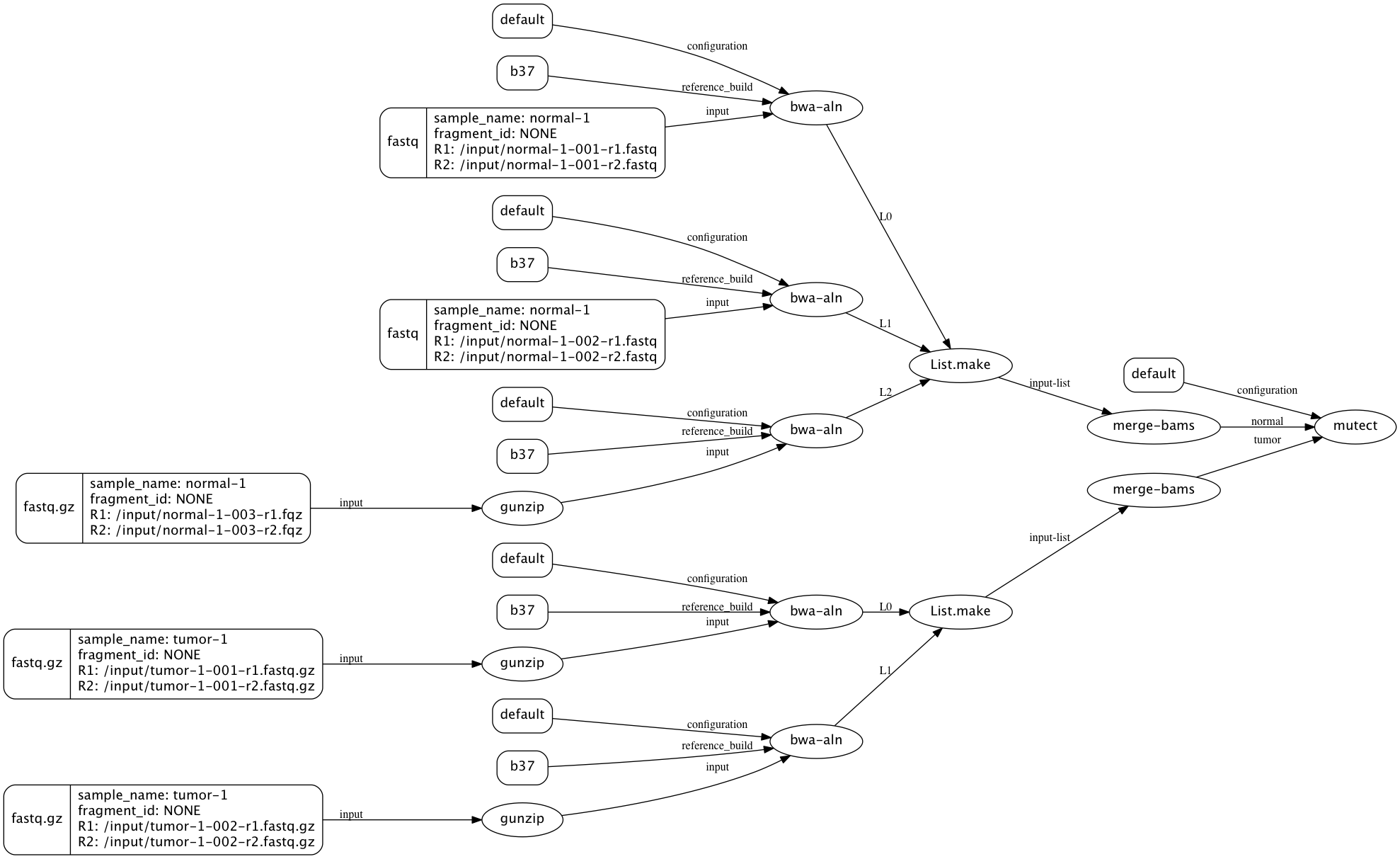### Epidisco

Big (family of) pipeline(s) that drive a clinical trial and other people's analyses:
`hammerlab/epidisco/`

Cf. output to `dot`-graphs:We actually do extend the EDSL:

• Custom HTML “report.”
• Custom “saving” of important artifacts.

### Zoom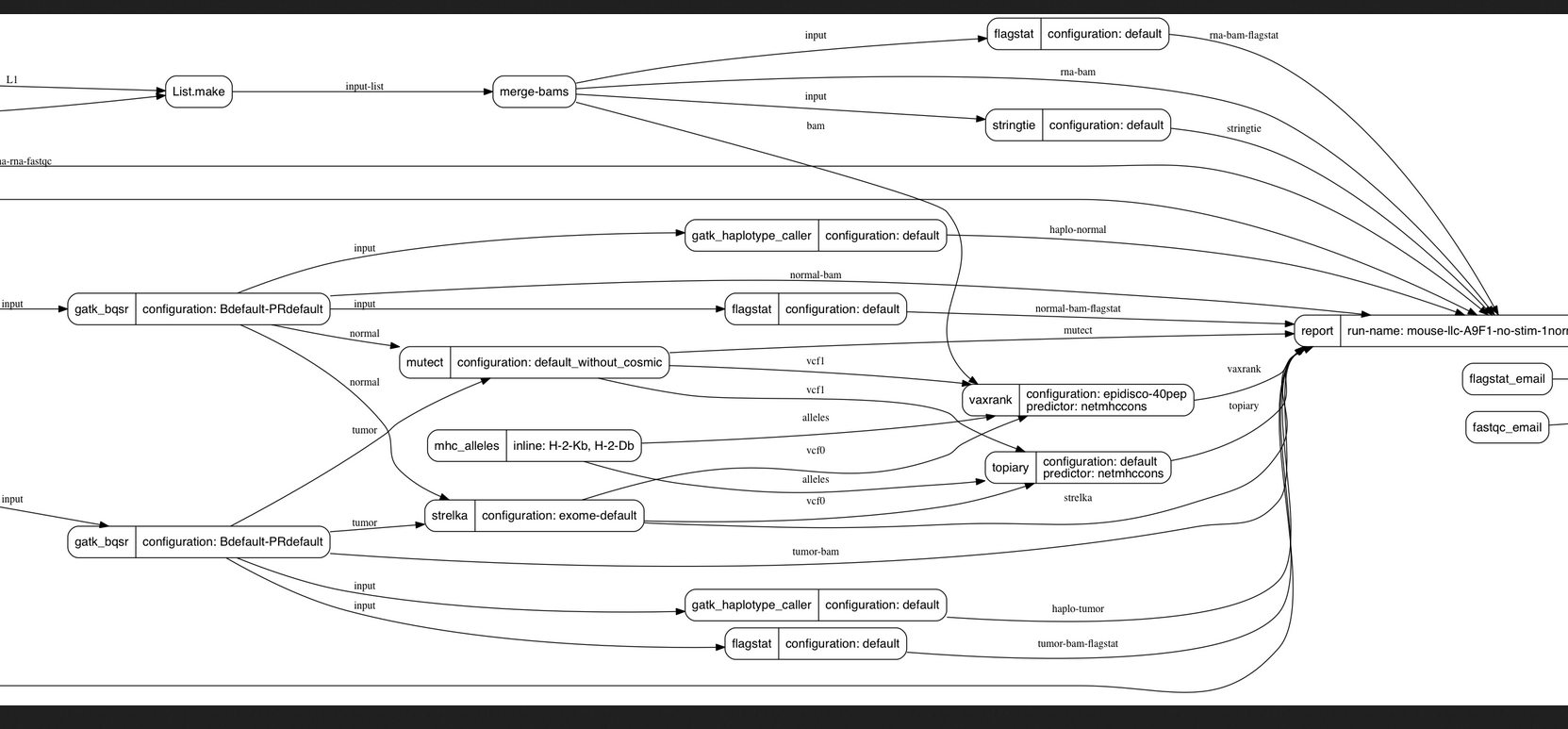### Deal With Insanity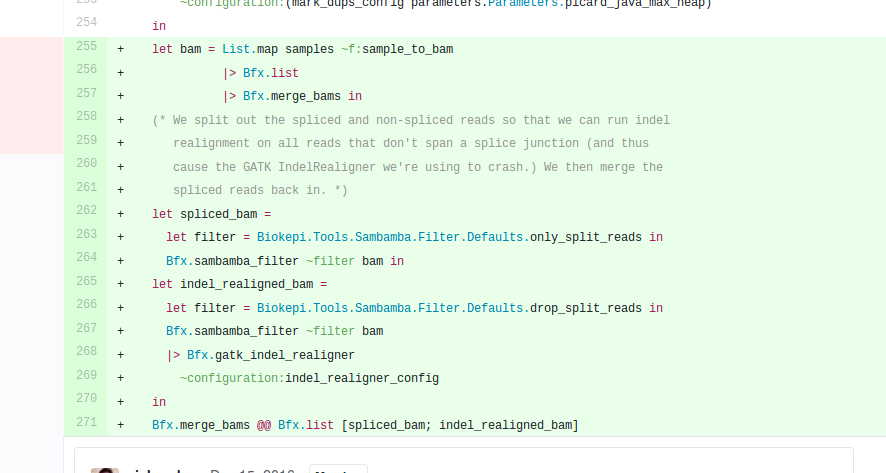### Limitations

Minor issues:

• Applying functors, while conceptually simple, scares beginners.
• Losing type variance because of the optimization framework.
• And in our case optimization framework is useful only for display.
• Cannot always use sub-modules because of `include`.
• Hence the flat/tagged API with `list_map`, `pair_first`, `pair_second`, …

Questions?